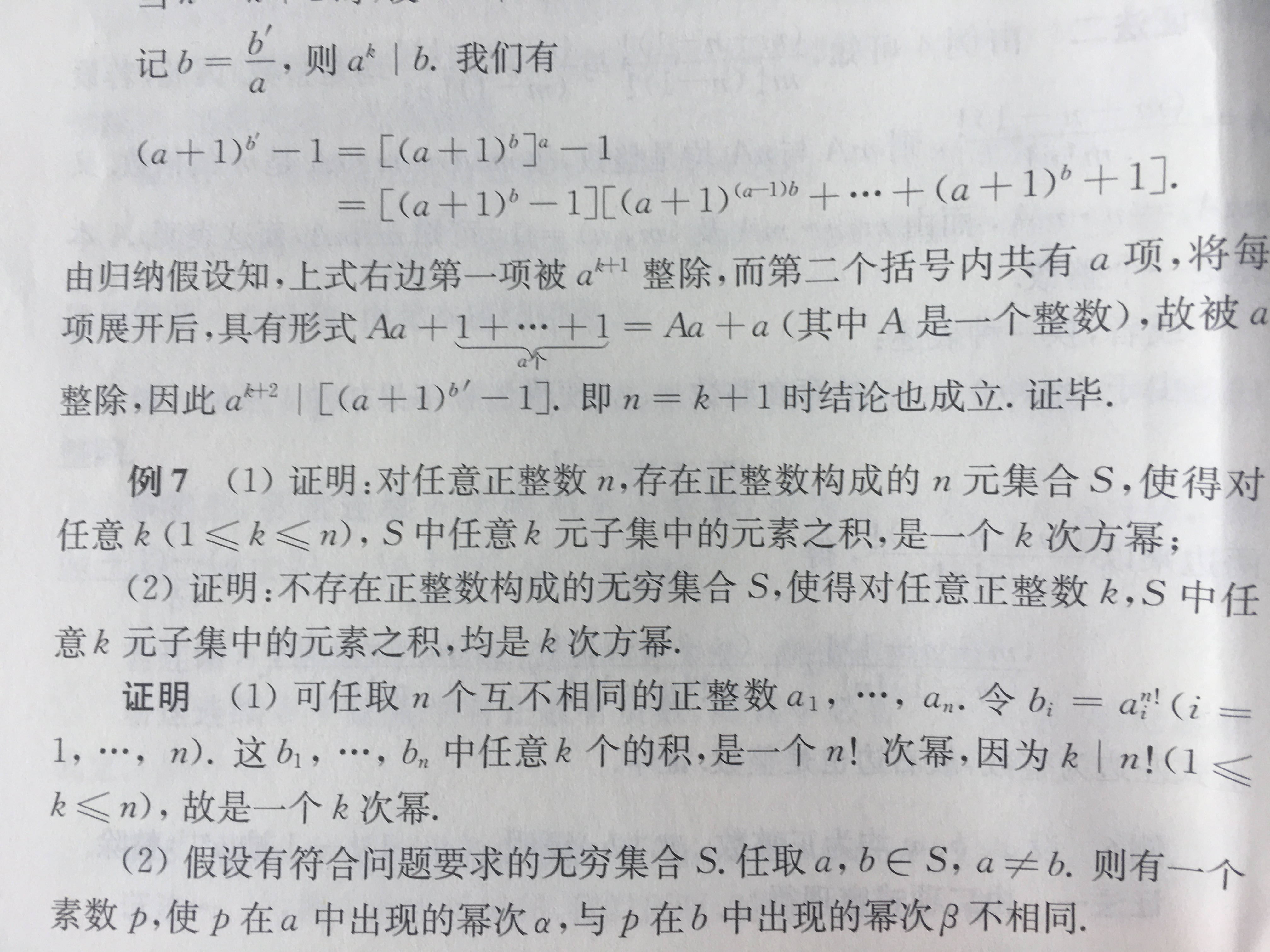# Why can a proposition hold for any given integer-sized set but not for infinite set?

I am a high school student and came across this problem on Number Theory.

(1) Prove that for any given positive integer $$n$$, there exists a set $$S \subseteq \mathbb{N^{*}}$$, such that for all $$1\le k \le n$$, any subset $$T$$ of $$S$$ of size $$k$$, satisfies $$\displaystyle \prod_{x\in T}x=t_x^k,t_x \in \mathbb{N}$$.

(2) Prove that it is impossible to find an infitite set $$S \subseteq \mathbb{N^{*}}$$, such that for all positive integer $$k$$, any subset $$T$$ of $$S$$ of size $$k$$ satisfies $$\displaystyle \prod_{x\in T}x=t_x^k,t_x \in \mathbb{N}$$.

Note that $$t_x$$ can be different for diffrent $$x$$. Or as in Chinese, 任意 $$k$$ 元子集之积都是 $$k$$ 次方幂。

I can easily give a construction of the first question, which is to arbitrarily choose $$n$$ integers $$a_1, a_2, \ldots, a_n$$, and let $$S=\{a_1^{n!}, a_2^{n!}, \ldots, a_n^{n!}\}$$. However, I just cannot understand why I can find such a set of arbitrary size but cannot find such a set of infinite size.

Can anyone explain why to me in a high-schooler-understandable way?

update1: Chinese statement.• I don't think it's the size of $S$ that makes the big difference (you could easily modify your solutino to (1) to let $S$ be infinite), but rather than in (2) one needs to handle infinitely many exponents in $t^k$. (The assumption that $S$ be infinite in (2) isn't necessary to state because it's obvious that a finite $S$ doesn't contain subsets of size $k$ for all $k\in \Bbb N$.) Aug 28, 2021 at 3:39
• That being said, I'm not sure (2) is accurate: it seems that the example $S=\{1^1, \; 2^2, 3^2,\; 4^3, 5^3, 6^3, \; 7^4, 8^4, 9^4, 10^4, \; \dots \}$ works. Aug 28, 2021 at 3:41
• Sorry, I mis-translated! It should be any subset of size $k$ Aug 28, 2021 at 3:43
• @GregMartin I have edited the question a bit and clarified something. It's hard to write mathematical-English really. Aug 28, 2021 at 3:49
• Technically, the property is true for an infinite set, if you still only have the property for $1\leq k\leq n.$ It’s only when you cosider the size of $S$ and the maximum for $k$ as the same that it fails. Aug 28, 2021 at 3:49